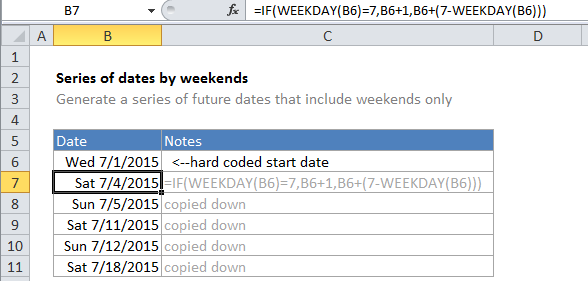## Excel Office

Excel How Tos, Tutorials, Tips & Tricks, Shortcuts

# Generate series of dates by weekends in Excel

This tutorials covers how to generates Series of dates increment by weekends from a single start date in Excel.

If need to generate a dynamic series of dates with a formula that include only future weekend dates (i.e. Sat and Sun), you can do so with a formula that uses the IF and WEEKDAY functions.

## Formula

`=IF(WEEKDAY(date)=7,date+1,date+(7-WEEKDAY(date)))`## Explanation

Worked Example:   If cell equals in Excel

In the example, B6 is the hard-coded start date and the formula in B7 is:

`=IF(WEEKDAY(B6)=7,B6+1,B6+(7-WEEKDAY(B6)))`

To solve this formula, Excel first calculates the weekday value for the date in B6. By default, weekday will return 1 for Sunday and 7 for Saturday. Next, Excel tests the weekday inside the IF statement, using B6=7 as the logical test. If B6 = 7, the date in B6 is a Saturday and the result if true is returned: B6 + 1. So, if B6 is a Saturday, the formula returns the next day (a Sunday).

Worked Example:   Excel If, Nested If, And/Or Criteria Examples

If not, the result if false is returned:

`B6+(7-WEEKDAY(B6))`

To solve this part of the formula, Excel calculates the weekday value of B6, then subtracts that value from 7. The result is added to B6. So, for Monday through Friday, this looks like this:

Worked Example:   Invoice status with nested if in Excel

B6+(7-2) = B6+5 <– Mon
B6+(7-3) = B6+4 <– Tue
B6+(7-4) = B6+3 <– Wed
B6+(7-5) = B6+2 <– Thu
B6+(7-6) = B6+1 <– Fri

Note: you’ll need to supply a date at least one day before the first Saturday you want to generate.